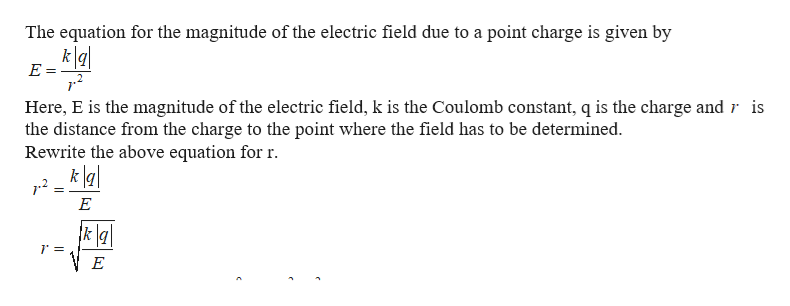# A particle has a charge of -6.10 nC .At what distance from this particle does its electric field have a magnitude of 12.0 N/C ?Express your answer with the appropriate units.

Question
47 views

A particle has a charge of -6.10 nC .

At what distance from this particle does its electric field have a magnitude of 12.0 N/C ?
check_circle

Step 1help_outlineImage TranscriptioncloseThe equation for the magnitude of the electric field due to a point charge is given by k g E = Here, E is the magnitude of the electric field, k is the Coulomb constant, q is the charge andr is the distance from the charge to the point where the field has to be determined Rewrite the above equation for r k a E k \a\ E fullscreen

### Want to see the full answer?

See Solution

#### Want to see this answer and more?

Solutions are written by subject experts who are available 24/7. Questions are typically answered within 1 hour.*

See Solution
*Response times may vary by subject and question.
Tagged in

### Physics